# 51

By Peter Mühlbacher, Research Scientist at Metaculus, and Peter Scoblic, Director of Nuclear Risk at Metaculus

Metaculus is a forecasting platform where an active community of thousands of forecasters regularly make probabilistic predictions on topics of interest ranging from scientific progress to geopolitics. Forecasts are aggregated into a time-weighted median, the “Community Prediction”, as well as the more sophisticated “Metaculus Prediction”, which weights forecasts based on past performance and extremises in order to compensate for systematic human cognitive biases. Although we feature questions on a wide range of topics, Metaculus focuses on issues of artificial intelligence, biosecurity, climate change and nuclear risk.

In this post, we report the results of a recent analysis we conducted exploring the performance of all AI-related forecasts on the Metaculus platform, including an investigation of the factors that enhance or degrade accuracy.

Most significantly, in this analysis we found that both the Community and Metaculus Predictions robustly outperform naïve baselines. The recent claim that performance on binary questions is “near chance” requires sampling on only a small subset of the forecasting questions we have posed or on the questionable proposition that a Brier score of 0.207 is akin to a coin flip. What’s more, forecasters performed better on continuous questions, as measured by the continuous ranked probability score (CRPS). In sum, both the Community Prediction and the Metaculus Prediction—on both binary and continuous questions—provide a clear and useful insight into the future of artificial intelligence, despite not being “perfect”.

# Summary Findings

We reviewed Metaculus’s resolved binary questions (“What is the probability that X will happen?”) and resolved continuous questions (“What will be the value of X?”) that were related to the future of artificial intelligence. For the purpose of this analysis, we defined AI-related questions as those which belonged to one or more of the following categories: “Computer Science: AI and Machine Learning”; “Computing: Artificial Intelligence”; “Computing: AI”; and “Series: Forecasting AI Progress.” This gave us: 64 resolved binary questions (with 10,497 forecasts by 2,052 users) and 88 resolved continuous questions (with 13,683 predictions by 1,114 users). Our review of these forecasts found:

• Both the community and Metaculus predictions robustly outperform naïve baselines.
• Analysis showing that the community prediction’s Brier score on binary questions is 0.237 relies on sampling only a small subset of our AI-related questions.
• Our analysis of all binary AI-related questions finds that the score is actually 0.207 (a point a recent analysis agrees with), which is significantly better than “chance”.
• Forecasters performed better on continuous questions than binary ones.

# Top-Line Results

This chart details the performance of both the Community and Metaculus predictions on binary and continuous questions. Please note that, for all scores, lower is better and that Brier scores, which range from 0 to 1 (where 0 represents oracular omniscience and 1 represents complete anticipatory failure) are roughly comparable to continuous ranked probability scores (CRPS) given the way we conducted our analysis. (For more on scoring methodology, see below.)

# Results for Binary Questions

We can use Brier scores to measure the quality of a forecast on binary questions. Given that a Brier score is the mean squared error of a forecast, the following things are true:

1. If you already know the outcome, you’ll get a Brier score of 0 (great!).
2. If you have no idea, you really should predict 50%. This always gives a Brier score of 0.25.
3. If you have no idea, but think that you do, i.e. you submit (uniformly) random predictions, you’ll get a Brier score of 0.33 in expectation, regardless of the outcome.

So, how do we judge the value of a Brier score of 0.207? Is it fair to say that it is close to “having no idea”?

No. Here’s why. Let’s assume for a moment that your forecasts are perfectly calibrated. In other words, if you say something happens with probability p, it actually happens with probability p. We can map the relationship between any question whose “true probability” is p and the Brier score you would receive for forecasting that the probability is p, giving us a graph like this: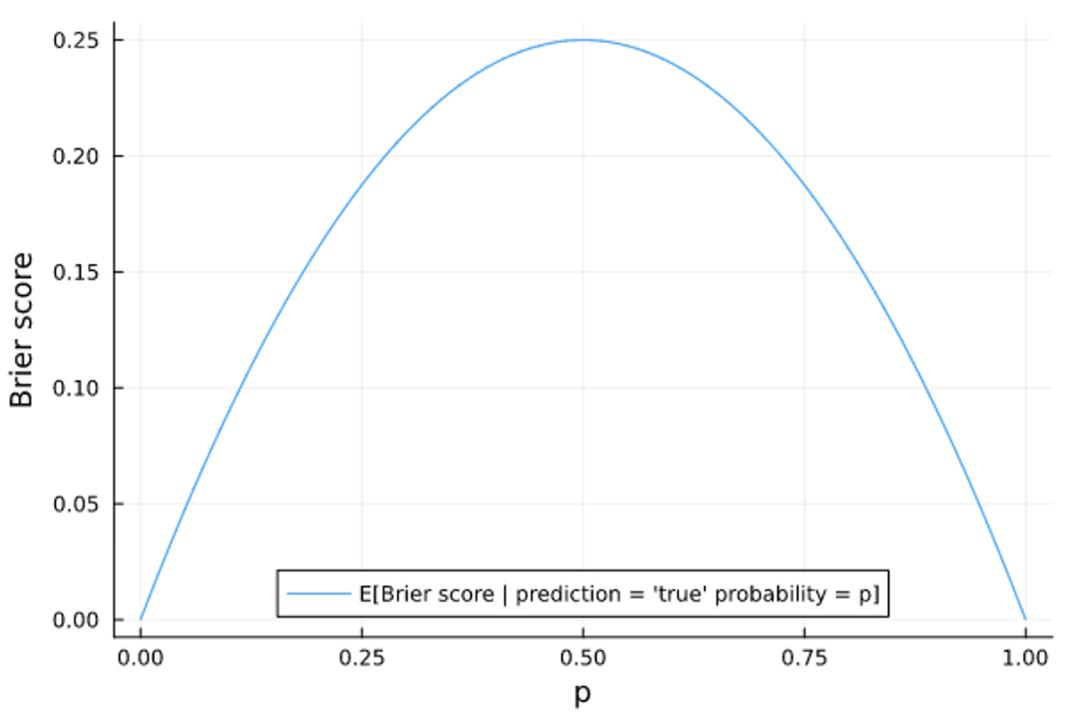This shows that even a perfectly calibrated forecaster will achieve a Brier score worse than 0.207 when the true probability of a question is between 30% and 70%. So, to achieve an overall Brier score better than 0.207, one would have to have forecast on a reasonable number of questions whose true probability was less 29% or greater than 71%. In other words, even a perfectly calibrated forecaster could wind up with a Brier score near 0.25, depending on the true probability of the questions they were predicting. So, assuming a sufficient number of questions, the idea that one could get a Brier score of 0.207 simply by chance is untenable. Remember: predicting 50% on every question would give you a Brier score of 0.25 (which is 19% worse) and random guessing would give you a Brier score of 0.33 (which is 57% worse).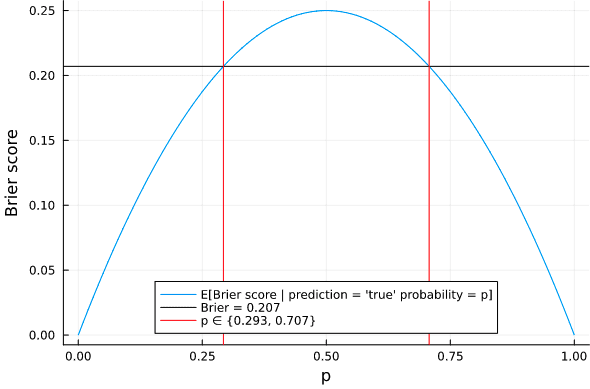Metaculus makes no claim that the Community Prediction is perfectly calibrated, but neither do we have enough information to claim that it is not well-calibrated. Using 50% confidence intervals for the Community Prediction’s “true probability” (given the fraction of questions resolving positively), we find that about half of them intersect the y=x line, which indicates perfect calibration: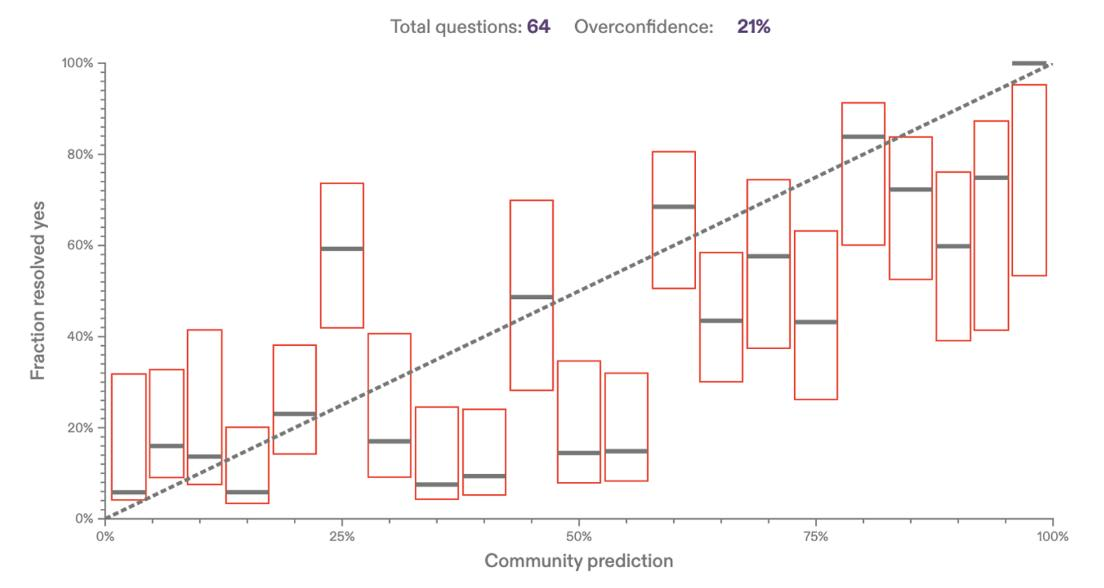A simulation can help us understand what Brier scores to expect and how much they would fluctuate on our set of 64 binary AI questions if we assumed each to resolve independently as 'Yes' with probability equal to its average Community Prediction. Resampling outcomes repeatedly, we get the following distribution, which shows that even if the (average) Community Prediction was perfectly calibrated, it would get a Brier score worse than 0.207 nearly a quarter of the time: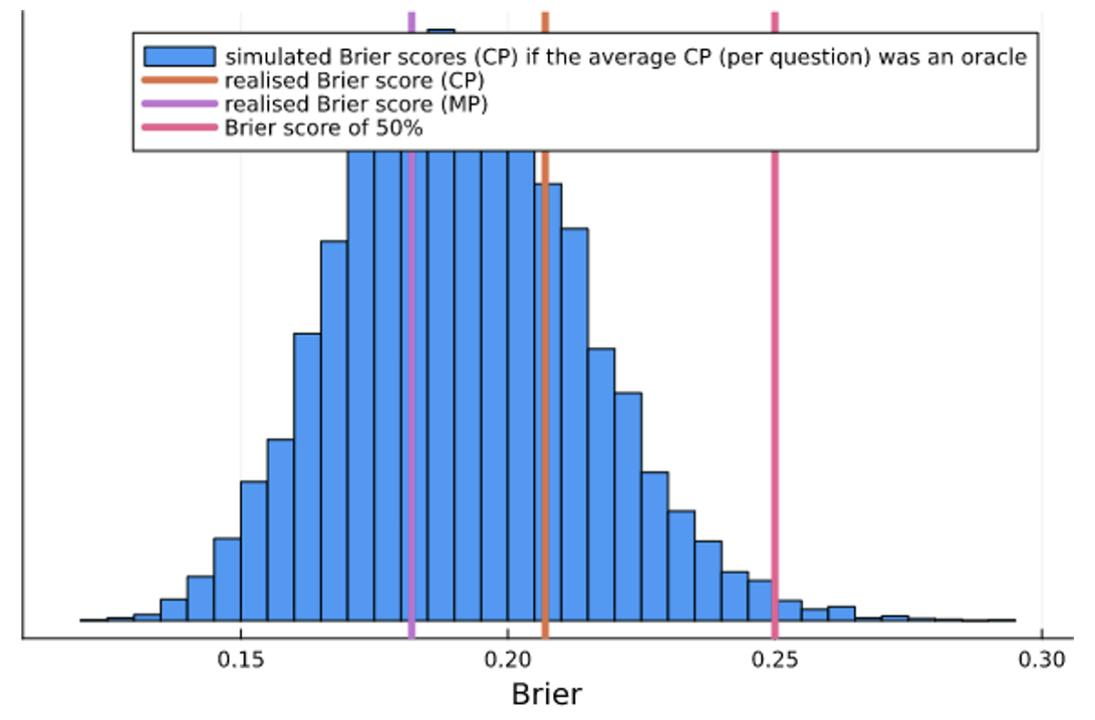If we don’t have enough data to reject the hypothesis that the community prediction is perfectly calibrated, then we certainly cannot conclude that “the community prediction is near chance.” This analysis in no way suggests that the Community Prediction is perfectly calibrated or that it is the best it could be. It simply illustrates that a Brier score of 0.207 over 64 questions is far better than “near chance,” especially when we consider that forecasting performance is partly a function of question difficulty. We suspect that AI-related questions tend to be intrinsically harder than many other questions, reinforcing the utility of the Community Prediction. The Metaculus Prediction of 0.182 is superior.

# Results for Continuous Questions

Many of the most meaningful AI questions on Metaculus require answers in the form of a continuous range of values, such as, “When will the first general AI system be devised, tested, and publicly announced?” We assessed the accuracy of continuous forecasts, finding that the Community and Metaculus predictions for continuous questions robustly outperform naïve baselines. Just as predictions on binary questions should outperform simply predicting 50% (which yields a Brier score of 0.25), predictions on continuous questions should outperform simply predicting a uniform distribution of possible outcomes (which yields a CRPS of 0.172 on the questions in this analysis).

Here, again, both the Community Prediction (0.096) and the Metaculus Prediction (0.103) were significantly better than baseline. In fact, the Community and Metaculus predictions performed considerably better on continuous questions than on binary questions. We can bootstrap the set of resolved questions to simulate how much scores could fluctuate, and we find that the fluctuations would have to conspire against us in the most unfortunate possible way (p<0.1%) to achieve even the baseline you’d get by predicting a uniform distribution. As we can see from the histograms below, it is more difficult for luck to account for a CRPS better than baseline than it is for a Brier score. So, if we cannot say that a Brier score of 0.207 is near chance, we certainly cannot say that a CRPS of 0.096 is near chance.

#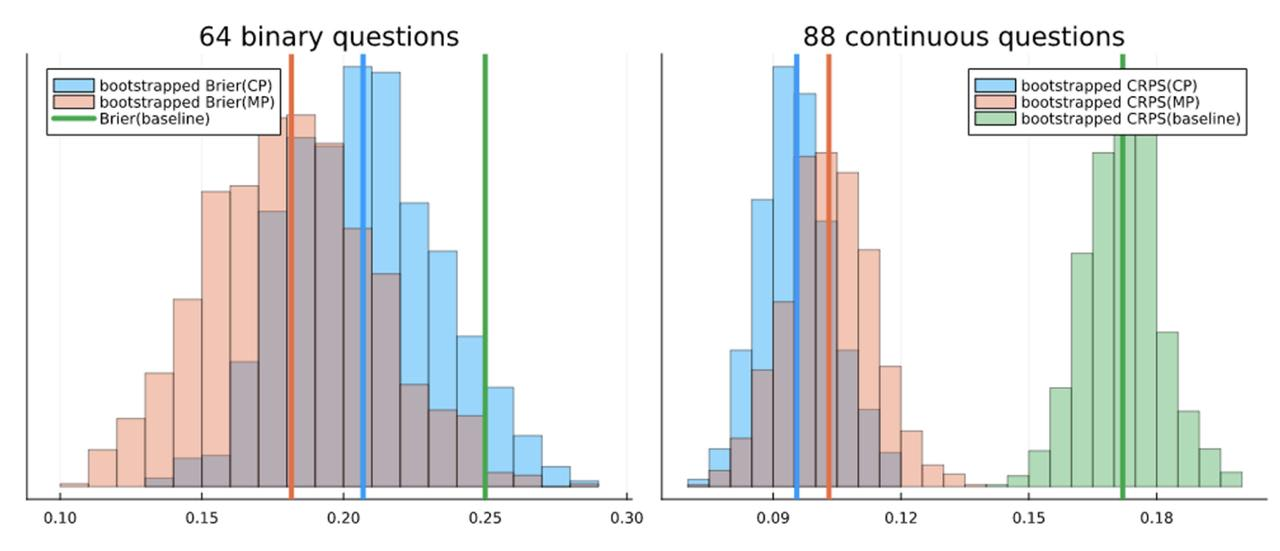Limitations and Directions for Further Research

Metaculus asks a wide range of questions related to artificial intelligence, some of which are more tightly coupled to A(G)I timelines than others. The AI categories cover a wide range of subjects, including:

• AI capabilities, such as the development of weak general AI;
• Financial aspects, like funding for AI labs;
• Legislative matters, such as the passing of AI-related laws;
• Organizational aspects, including company strategies;
• Meta-academic topics, like the popularity of research fields;
• Societal issues, such as AI adoption;
• Political matters, like export bans;
• Technical questions about required hardware.

Being fundamentally mistaken about fundamental drivers of AI progress, like hardware access, can impact the accuracy of forecasts for more decision-relevant questions, like the timing of developing AGI. While accurate knowledge of these issues is necessary for reliable forecasts in all but the very long term, it might not be sufficient. In other words, a good track record across all these questions doesn't guarantee that predictions on any specific AI question will be accurate. The optimal reference class for evaluating forecasting track records is still an open question, but for now, this is the best option we have.

Forecaster Charles Dillon has also grouped questions to explore whether Metaculus tends to be overly optimistic or pessimistic regarding AI timelines and capabilities. Although we haven't had enough resolved questions since his analysis to determine if his conclusions have changed, his work complements this study nicely. We plan to perform additional forecast accuracy analyses in the future.

# Methodological Appendix

## Scoring rules for predictions on binary questions

All scoring rules below are chosen such that lower = better.

All scoring rules below are strictly proper scoring rules, i.e. predicting your true beliefs gets you the best score in expectation.

The Brier score for a prediction  on a binary question with outcome  is . So

• an omniscient forecaster, perfectly predicting every outcome, will thus get a Brier score of 0,
• a completely ignorant forecaster, always predicting 50%, will get a Brier score of ,
• a forecaster guessing (uniformly) random probabilities will get a Brier score of  on average.

An average Brier score higher than 0.25 means that we’re better off just predicting 50% on every question.

## Scoring rules for predictions on continuous questions

On Metaculus, forecasts on continuous questions are submitted in the form of

1. a function  on a compact interval, together with
2. a probability  for the resolution to be below the lower bound,
3. a probability  for the resolution to be above the upper bound,

such that .

Some questions (all older questions) have “closed bounds”, i.e. they are formulated in a way that the outcome cannot be below the lower bound () or above the upper bound (). Newer questions can have any of the four combinations of a closed/open lower bound and a closed/open upper bound.

For the analysis it is convenient to shift and rescale bounds & outcomes such that outcomes within bounds are in .

## CRPS

The Continuous Ranked Probability Score for a prediction on a continuous question with outcome  is given by . This is equivalent to averaging the Brier score of all (implicitly defined by the CDF) binary predictions of the form , which allows us to compare continuous questions with binary questions.

## Scoring a time series of predictions

Given

• a scoring rule ,
• a time series of predictions ,
• where  are the time of the first forecast and the close time after which no predictions can be submitted anymore, respectively,
• and the outcome ,

we define the score of  to be

.

This is just a time-weighted average of the scores at each point in time.

Concretely, if the first prediction arrives at time , the second prediction arrives at time , and the question closes at , then the score is  times the score of the first prediction and  times the score of the second prediction because the second prediction was “active” twice as long.

Metaculus is an online forecasting platform and aggregation engine working to improve human reasoning and coordination on topics of global importance. By bringing together an international community and keeping score for thousands of forecasters, Metaculus is able to deliver machine learning-optimized aggregate predictions that both help partners make decisions and benefit the broader public.

# Reactions

Sorted by Click to highlight new comments since:

I am glad you did this. Thanks!

It is interesting that Metaculus' community predictions are 7.29 % (= 0.103/0.096) more accurate than Metaculus' predictions according to CRPS (continuous question). In contrast, based on the log score, Metaculus' community predictions are 13.1 % (= 0.842/0.969) worse.

Do you have any thoughts on whether CRPS is preferable to log score? Intuitively, CRPS seems better because it relies on more information about the forecast. The log score only uses the probability density at the resolved values, whereas CRPS taken into account the whole CDF. I think it would be nice to add the CRPS to Metaculus' track record page.

This shows that even a perfectly calibrated forecaster will achieve a Brier score worse than 0.207 when the true probability of a question is between 30% and 70%.

This is probably a nitpick, but I am not sure I agree with your framing of "true probability". Even for a coin flip, where one usually says there is a 50 % chance of heads/tails, the true probability of heads/tails will be 0 or 1, in the sense that in theory one could predict the outcome with near certainty having all the relevant information. So I think I would prefer the term resilient probability, i.e. one that is unlikely to be updated further in response to new information (in the same way that one is unlikely to update away from 50/50 in a coin flip).

• For continuous questions we have that the Community Prediction (CP) is roughly on par with the Metaculus Prediction (MP) in terms of CRPS, but the CP fares better than the MP in terms of (continuous) log-score. Unfortunately the "higher = better" convention is used for the log score on the track record page—note that this is not the case for Brier or CRPS, where lower = better.
This difference is primarily due to the log-score punishing overconfidence more harshly than the CRPS: The CRPS (as computed here) is bounded between 0 and 1, while the (continuous) log-score is bounded "in the good direction", but can be arbitrarily bad. And, indeed, looking at the worst performing continuous AI questions shows that the CP was overconfident which is further exacerbated when extremising. This hardly matters if the CRPS is already pretty bad, but it can matter a lot for the log-score.
This is not just anecdotal evidence, you can check this yourself on our track record page, filtering for (continuous) AI questions, and checking the "surprisal function" in the "continuous calibration" tab.
• > Do you have any thoughts on whether CRPS is preferable to log score?
Too many! Most are really about the tradeoffs between local and non-local scoring rules for continuous questions. (Very brief summary: There are so many tradeoffs! Local scoring rules like the log-score have more appealing theoretical properties, but in practice they likely add noise. Personally, I also find local scoring rules more intuitive, but most forecasters seem to disagree.)
• I see where you're coming from with the "true probability" issue. To be honest I don't think there is a significant disagreement here. I agree it's a somewhat silly term—that's why I kept wrapping it in scare quotes—but I think (/hoped) it should be clear from context what is meant by it. (I'm pretty sure you got it, so yay!)
Overall, I still prefer to use "true probability" over "resilient probability" because a) I had to look it up, so I assume a few other readers would have to do the same and b) this just opens another can of worms: Now we have to specify what information can be reasonably obtained ("what about the exact initial conditions of the coin flip?", etc.) in order to avoid a vacuous definition that renders everything bar 0 and 1 "not resilient".
I'm open to changing my mind though, especially if lots of people interpret this the wrong way.

Thanks for the clarifications!

Unfortunately the "higher = better" convention is used for the log score on the track record page—note that this is not the case for Brier or CRPS, where lower = better.

I have corrected my initial comment (using strikethrough like this).

Is the dataset and analysis available to download anywhere? It would be great if your work can be independently reproduced! (Not meaning to imply any mistakes but there is an obvious conflict of interest here.)

You may want to have a look at our API!

As for the code, I wrote it in Julia and as part of a much bigger, ongoing project, so it's a bit of a mess. I.e. lots of code that's not relevant for this particular analysis. If you're interested, I could either send it to you directly or make it more public after cleaning it up a little.

Curated and popular this week
Recent opportunities
165
· 7d ago · 1m read